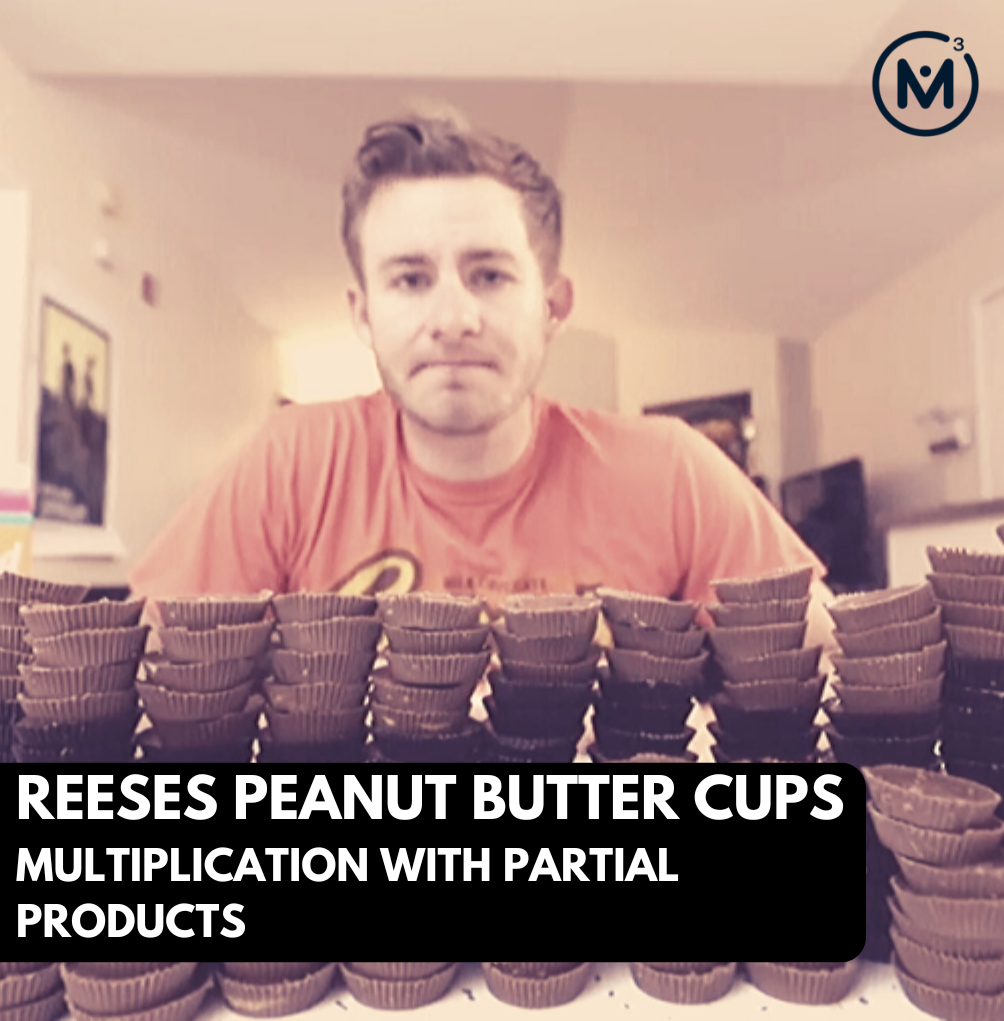## REESES PEANUT BUTTER CUPS​ [DAY 2]

### Multiplication with partial products

Decompose an array to reveal partial products in order to estimate a large quantity.

## Intentionality & Unit Overview### Access each lesson from this unit using the navigation links below

Students will determine quantities using arrays. They will be encouraged to explore partial products.

The purpose of the Day 2 activities is to reinforce key concepts from Day 1. Students will engage in a string of related problems through a math talk and will have an opportunity to complete independent purposeful practice. The math talk and purposeful practice serve to develop a deeper understanding of the following big ideas.

• Numbers can be decomposed.
• The relationship between repeated addition and multiplication.
• The relationships between rows and columns in an array.
• The distributive property of multiplication over addition and over subtraction.
• Numbers can be decomposed
• The relationship between repeated addition and multiplication
• The relationships between rows and columns in an array
• The distributive property of multiplication over addition and over subtraction.

## Math Talk

The purpose of today’s math talk is to explore the identity property of subtraction (subtracting 0 from a number does not change the value 4-0=4) and near numbers. It is important for students to recognize how numbers are related to each other. When students are counting, they do not necessarily think about the relationship between numbers, but by recognizing near numbers, it may help students with minus 1 (-1) or minus 2 (-2) facts.

Identity property:

Students need to generalize the idea that when taking away 0 from any number, we are left with the same amount because nothing was taken away. It seems like a concept that could be glossed over but it may be a source of confusion for some students.

## Purposeful Practice

Login/Join to access the entire Teacher Guide, downloadable slide decks and printable handouts for this lesson and all problem based units.